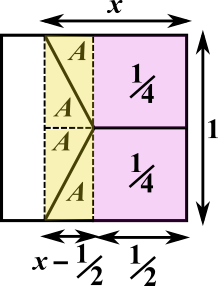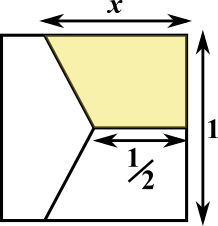#### You may also like### Hallway Borders

What are the possible dimensions of a rectangular hallway if the number of tiles around the perimeter is exactly half the total number of tiles?### Square Pegs

Which is a better fit, a square peg in a round hole or a round peg in a square hole?### Boxed In

A box has faces with areas 3, 12 and 25 square centimetres. What is the volume of the box?

# Dividing a Square

##### Age 11 to 14 ShortChallenge Level
Splitting the square into rectangles and triangles
Using a vertical line to break each parallelogram into a square and a triangle, and another vertical line to break the pentagon into a rectangle and a triangle, lots of congruent shapes emerge, as shown below.

The areas of the purple squares are $\tfrac{1}{4}$, because the horizontal distance from the centre to the edge of the large square if $\tfrac{1}{2}$ and half of the side length is $\frac{1}{2}$, and $\frac{1}{2}\times\frac{1}{2}=\frac{1}{4}$. The four triangles are all congruent and their areas have been labelled $A$.The area of one of the trapezia is $\frac{1}{4}+A$, so $\frac{1}{4}+A=\frac{1}{3}$, so $A=\frac{1}{3}-\frac{1}{4}=\frac{1}{12}$

The yellow area is $1\times\left(x-\frac{1}{2}\right)=x-\frac{1}{2}$, so $4A=x-\frac{1}{2}$. \begin{align}&4A=\tfrac{4}{12}=\tfrac{1}{3}\\ \Rightarrow&\tfrac{1}{3}=x-\tfrac{1}{2}\\ \Rightarrow&x=\tfrac{1}{3}+\tfrac{1}{2}=\tfrac{5}{6}\end{align}

Using the formula for the area of a trapezium
The area of the trapezium coloured yellow in the diagram below is $\frac{1}{2}\times(a+b)\times h=\frac{1}{2}\times\left(x+\frac{1}{2}\right)\times\frac{1}{2}=\frac{1}{4}x+\frac{1}{8}$.So $\frac{1}{4}x+\frac{1}{8}=\frac{1}{3}$, so $\frac{1}{4}x=\frac{1}{3}-\frac{1}{8}$, so $x=\frac{4}{3}-\frac{1}{2}=\frac{5}{6}$.
You can find more short problems, arranged by curriculum topic, in our short problems collection.